﻿ Experimental Assessment of Noise Generation from I.C. Engine Intake and Exhaust Systems Components

### Experimental Assessment of Noise Generation from I.C. Engine Intake and Exhaust Systems Components

Sabry AllamOPEN ACCESSPEER-REVIEWED

## Experimental Assessment of Noise Generation from I.C. Engine Intake and Exhaust Systems Components

Automotive Technology Department, Faculty of Industrial Education, Helwan University, Cairo, Egypt

### Abstract

Several acoustic elements are used in internal combustion engine to tune engine intake/exhaust manifold systems. Components in intake and exhaust systems that create flow separation can for certain conditions and frequencies amplify incident sound waves. This vortex-sound phenomena is the origin for whistling, i.e., the production of tonal sound at frequencies close to the resonances of a duct system. One way of predicting whistling potential is to compute the acoustic power balance, i.e., the difference between incident and scattered sound power. This can readily obtained if the scattering matrix is known for the object. For the low frequency plane wave case this implies knowledge of the two-port data, which can be obtained by numerical and experimental methods. In this paper the development of multi-port models to describe linear acoustic problems in ducts with flow is presented. From an engineering point of view this field covers many important applications ranging from ventilation ducts in vehicles or buildings to intake/exhaust ducts on IC engines and power plants.In this paper the procedure to experimentally determine whistling potential will be presented and applied to side-branch resonators and orifices.

### At a glance: Figures

12345
Prev Next

• Allam, Sabry. "Experimental Assessment of Noise Generation from I.C. Engine Intake and Exhaust Systems Components." American Journal of Vehicle Design 3.1 (2015): 6-15.
• Allam, S. (2015). Experimental Assessment of Noise Generation from I.C. Engine Intake and Exhaust Systems Components. American Journal of Vehicle Design, 3(1), 6-15.
• Allam, Sabry. "Experimental Assessment of Noise Generation from I.C. Engine Intake and Exhaust Systems Components." American Journal of Vehicle Design 3, no. 1 (2015): 6-15.

 Import into BibTeX Import into EndNote Import into RefMan Import into RefWorks

### 1. Introduction

1.1. Background

Flow-acoustic interaction in flow duct systems could lead to intense noise, often denoted a whistle, which not only could be disturbing but also could lead to mechanical failure of the structure. Full simulations of a typical system such as a gas pipelines or automotive exhaust/intake systems are still too computationally expensive to be viable. A common simplification of the problem is to divide the system into a network of linear acoustic multi ports. Each of these “black boxes” could then be determined analytically, numerically or experimentally. This approach is widely used for studying passive system properties such as reflection and transmission of sound. For linear duct aeroacoustic problems, i.e., cases with linear wave propagation and sound generation uncoupled to the acoustic field, a multi-port represents the most general way of describing acoustic sources [1, 2]. Knowledge of the multi-port data for all active (fans, flow constrictions,...) and passive (straight ducts,...) elements in a duct system, plus the radiation impedances at duct openings, enables a complete acoustic analysis. This is of course important for engineering acoustics, but to make it useful experimental or numerical methods to determine multi-port data are needed. Since 1970 most of the works done in this field have been focusing on fluid machine applications, the low frequency 1D (plane) wave range and have been experimentally validated . The progress in Computational Fluid Mechanics (CFD) during the last 10-15 years has opened the possibility for the determination of both the passive and the active part via numerical methods. In particular the possibility to compute the active part is important. For fluid machines (IC-engines, compressors, fans,....) the periodic part of the spectrum can normally be obtained by so called U-RANS, but the broad-band part requires Large Eddy Simulations (LES) and is still not feasible to compute for 3D cases and realistic Reynolds numbers. An example of an area where 1-port source data determination via (1D) CFD codes today are common practice is the gas exchange analysis of IC-engines [3, 4, 5, 6].

During the last decade efforts to also apply multi-port models to study flow generated sound in ducts and thermo-acoustics have come into focus. Aurégan and Starobinski  proposed an approach that provides indication for the dissipation or amplification of sound in a multi-port system. Åbom et. al.  investigated experimentally both the active and passive two-port data for orifice plates. De Roeck et. al.  investigated expansion chamber mufflers and both measured and computed the two-port data. For the active part a 2D compressible LES model was used and the analysis restricted to sound produced by low frequency Rossiter modes. The passive part or the scattering matrix was computed using linearized Euler equations (LEE). Testud et.al.  investigated sound amplification and whistling for orifices using experimental two-port data. Sattelmayer and Polifke  investigated thermo-acoustic instabilities in combustors modelled as two-port sources. Karlsson and Åbom  studied a T-junction using experimentally determined three-port data.

Recently, Kierkegaard et al.  demonstrated a linear aeroacoustic simulation methodology to predict the whistling of an orifice plate in a flow duct. The methodology is based on a linearized Navier–Stokes solver  in the frequency domain with the mean flow field taken from a Reynolds-Averaged Navier–Stokes (RANS) solution. The whistling potentiality is investigated via an acoustic energy balance  for the in-duct element and good agreement with experimental data is shown.

The resonator is an ideal, simple acoustic device that has applications in acoustic systems. In acoustic system design, the Helmholtz Resonator (HR) and Side branch resonator (SR) are often used to modify the acoustic response. For example, internal combustion engine intake manifold systems are designed so that the acoustic response enhances the engine performance, increases fuel economy and reduces emissions. When design considerations such as space and material limitations cause the acoustic response to degrade engine performance and/or create excessive noise, the solution is often to add a designed HR or SR to the system, thus improving the response .

The use of HR or SR creates flow separation that can for certain conditions and frequencies amplify incident sound waves. This phenomenon is the origin for whistling, i.e., the production of tonal sound at frequencies close to the resonances of a duct system. This paper aims to present an experimental method to study the flow separation and whistling phenomenons in single and double orifices and newly designed SR based on the passive part of the scattering matrix which is determined using the two-source method . The last is a standard experimental method to characterize the acoustic performance of an acoustic device i.e., using the existing measured data to study flow separation and whistling phenomenons in any acoustic element.

1.2. Structure of the Paper

The paper is organized as follows. In Section 2, theoretical representation of the problem, sound amplification and existence of system instabilities are presented. Experimental setup, measuring procedure and test object definitions are presented in Section 3. Results of measured scattering matrix, power amplification and whistling potentiality of different test objects are presented in Section 4. Work summary, conclusion and proposed extension of the research as future work are presented in Section 5.

### 2. Theoretical Representation

An active acoustic multi-port is a linear and time invariant black box with N degrees of freedom, which in the frequency domain can be written as [1, 2, 3, 4, 5]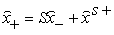(1)

where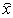is the state variable of choice, the plus and minus signs indicate travelling wave amplitudes out of (+) and in to (-) the multi-port respectively. Consequently the passive properties of the system, the reflection and transmission of sound, are represented by the scattering matrix S. The vector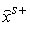represents the active part (sources) within the system which normally is assumed independent of the sound field; this is often a good assumption when modelling fluid machines. However, it is no problem to relax this assumption and include, e.g., vortex-sound interaction, by assuming that one part of the source strength can be modulated by incident acoustic waves. The source strength vectoris then split into two parts, one corresponding to the (linear) modulation given by the incident acoustic field while the other still represents the part independent of the acoustic field. The modulated part can be expressed as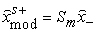(2)

which can be inserted into Eq.(1)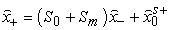(3)

where the subscript zero indicates the original scattering matrix and source vector, that is, the ones independent of the source coupling. Hence, a linear modulation of the source term simply adds to the scattering properties. This in turn implies that in an experimental characterization of amulti-port, information on acoustic source interaction effects is included in the scattering matrix representation, while the resulting source vector is always independent of the incident acoustic field. This conclusion is important since it means that by studying the scattering-matrix for a system it will be possible to determine if sound amplification occurs.

2.1. Sound Amplification

Now assuming that flow-acoustic interaction effects are included in the scattering matrix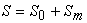the potential for a system to whistle can be examined by computing a power balance over the multi-port. Here a suitable state variable is the acoustic energy  which is simply related to the time averaged power as: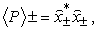(4)

where P now represents acoustic exergy. Now, denoting the scattering matrix related to exergy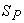, the time averaged acoustic power produced by a multi-port for a given incident acoustic field is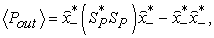(5)

where * is the Hermitian transpose. If for instance vortex-sound interaction results in an amplification of the incident sound this equation is positive.

One way of using this result is to assume excitation at only one port n at the time . Although a multi-port in practice is likely to experience incident sound at more than one port at the time this approach can give valuable insight into the systems characteristics. Normalizing the incident power at the port of interest to unity (that is,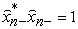) this can be expressed as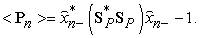(6)

A more generic approach was proposed by Aurégan and Starobinski .

The quadratic form in Eq. (5) is Hermitian and positive definite, and thus it can be transformed into a diagonal form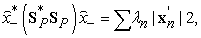(7)

where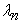are the real and positive eigenvalues of (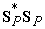) and the vector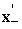is given by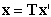. The transformation matrix T is a unitary matrix where the columns represent the eigenvectors of the Hermitian matrix (). Finally, inserting Eq.(7) into Eq.(6), the maximum/minimum time averaged acoustic power output can be expressed as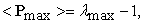(8)

and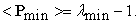(9)

That is, an eigenvalue larger than unity indicates amplification of the incoming perturbation. The actual relative excitation that yields a maximum (or minimum) can be found by studying the corresponding eigenvector.

2.2. Existence of System Instabilities (whistling)

The power balances described in the previous section indicates at which Strouhal number (St) ranges the given multi-port amplifies or attenuates incident acoustic waves. Now, the actual response to a given termination can be studied by introducing a reflection matrix R whose elements represent the passive and time invariant terminations of the system seen at each port [7, 15]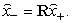(10)

Inserting Eq(10) into Eq.(1) yields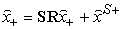(11)

From this the response (output) from the system can be solved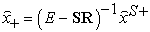(12)

Instabilities corresponding to exponentially growing harmonics correspond to poles for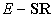in the lower (assuming the Fourier transform is defined by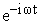complex half plane(Im(ω)<0). This formulation is in direct correspondence with the one used in control theory, where–SR is referred to as the open loop response. Here an alternative but equivalent formulation will beused and that is to investigate the system eigenfrequencies.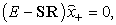(13)

where E is the unit matrix. This equation has non-trivial solutions (eigen frequencies) when where E is the unit matrix. This equation has non-trivial solutions (eigenfrequencies) when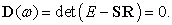(14)

If the system is reflection free R ≡ 0 and the function D will collapse to a single point (1,i0) in the complex D(ω)-plane.

There are various methods for finding the zeros of the complex function D(ω). One is to applyCauchy’s argument principle for complex functions. Assume that D(ω) is analytical (except at a finite number of points) in the complex plane ω. Now travelling around a contour in the ω-plane (at which D(ω) must be analytical) in the clockwise direction, D(ω) will encircle origo in the complex D(ω) plane in the same direction N times, where N is given by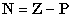(15)

Where Z and P stands for the zeros and poles of the function D(ω) inside the contour. Assuming that the system under study is casual and that D tends to 1 for large ω, implies that it is sufficient to study the variation of D(ω) along the real ω axis. Because the causality implies that D is analytical in the lower half plane for sufficiently large ω and will approach 1, i.e., the same value (= point) reached along the real axis for large ω. Also since roots and poles will appear in pairs, i.e., for each root/pole with a positive real part there is a corresponding located at –ω*, it is sufficient to investigate D(ω) for positive real ω. If there are no poles the number of clockwise encirclements of origowill represent the number of zeros in the lower half plane, representing exponentially growing eigenfrequencies. This version of the argument principle can be seen as an extension of the so called Nyquist stability criterium to an N-DOF system. Similar to the traditional Nyquistcriterium an estimate of the eigenfrequencies (the real part) are found when the function D(ω) crosses the negativereal axis thus completing one encirclement. The distance to zero at this crossing can be seen as a measure of the growth rate of the instability .

The requirement that there are no poles in D in the lower plane implies that S and R must have no poles. Physically this implies that the equations: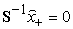and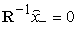has no zeros in the lower half plane, i.e., neither the multi-port nor the termination are whistling by themselves.

### 3. Tested Examples

3.1. Experimental Procedure

Experiments were carried out at room temperature using the flow acoustic test facility at the Marcus Wallenberg Laboratory (MWL) for Sound and Vibration research at KTH, which is represented in Figure 1. The test duct used during the experiments consisted of a standard steel-pipe with a wall thickness of 3 mm, duct inner diameter is varied according to test object; di=51 mm for single and double orifices, di=57 mm for resonators and overall length is around 7 meters.

The first test object was a single diaphragm orifice with a concentric hole and a diameter of do=32 mm and an orifice thickness of t = 8.5 mm, the second test object was a double diaphragm orifice with same dimensions, see Figure 2a and b. These two objects can be found in ventilation, IC-engines intake and exhaust systems for flow and pressure managements. The third, the fourth and fifth test objects are side branch resonators shown in Figure 2c, d and e. Six loudspeakers were used as external acoustic sources, and they were divided equally between the upstream and downstream side as shown in Figure 1. The distances between the loudspeakers were chosen to avoid any pressure minima at the source position. Six flush mounted condenser microphones (B&K 4938) were used, three upstream and three downstream of the test object for the plane wave decomposition. The microphone separations are chosen to fulfill the frequency ranges of interest up to 2 kHz. The two extra microphones up- and downstream denoted A and B are used to determine the active part , which is not addressed in this paper. All measurements are performed using the source switching technique , and the flow speed was measured upstream of the test section using a small pitot-tube connected to an electronic manometer at a distance of 1000 mm from the upstream loudspeakers section and the flow speeds were chosen to fit with the real application of each test object. More useful information and results for the first two test objects can be fined in Åbom et.al.  and .

Figure 1. Measurement configuration for plane wave decomposition at MWL.(a) Constructor drawing of the test rig that is used with Case 1 (single orifice) and Case 2 (double orifices), (b) Photo for the test rig with SR is connected
Figure 2. The test objects, (a) a single diaphragm orifice with a concentric hole with t/do=0.17 and do/di=0.63, (b) double orificest/do=0.17, di/do=0.63, L=3do (c)ovalshape resonator, (d) complex side branch resonator(e)two side-branches in series (compact resonator)
3.2. Passive Part

The passive part of the test object can be defined from the following two-port equation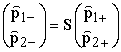(16)

where S is the scattering matrix. The passive part of the two-port is determined using the two-source method  where acoustic waves are excited at the inlet (case A) and, subsequently, at the outlet (case B). The scattering matrix is determined using the pressure levels at the sample positions, which are chosen at a certain distance up- and downstream of the orifice. The scattering matrix is calculated as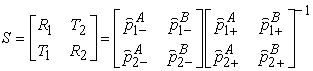(17)
3.3. Test Object Layout

The test objects as shown in Figure 2 can be break down into five acoustic elements that are usually used in real vehicle applications (a) a single diaphragm orifice with a concentric hole with orifice wall thickness, t=8.5 mm, t/do=0.17, do=51, is the duct diameter, (b)double orifices t/do=0.17, di/do=0.63, distance between two orifices L=3do, (c)oval shaped resonator, (d) complex side branch resonator and (e) two side-branches in series (compact resonator).

### 4. Results and Discussion

4.1. Single Orifice

As seen from Figure 3, a net (positive) amplification is obtained at the Strouhal –number St= 0.23, Here, St is computed in accordance with other investigations as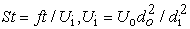(18)

where, f is frequency, t is the orifice thickness, d diameter (o for duct and i for orifice) U is the flow speed in the main duct, and Ui is the flow speed in the orifice. The maximum amplification depends on the flow speed and is approximately proportional to the Mach number in the orifice.

When < P > is larger than 0, the acoustic power output is larger than the input power (normalized to 1). Pmax based on Eq. (8) is the maximum possible output and Pmin based on Eq. (9) is the minimum. P1 and P2 (Eq. (6)) represent the output power for a unit power input (1) upstream or (2) downstream. As discussed in Ref.  the eigenvalue corresponding to the maximum amplification, corresponds to a velocity type of excitation at the orifice.

Figure 4. Plot of D(ω) in the complex plane for a system consisting of the tested orifice and free terminationup and downstream duct at M=0.0675

The computed behaviour for such a system represented by the complex valued plot of D(ω) (Eq. (14)) is shown in Figure 4. As seen in Figure 4, the system displays numerous encirclements around the base point (1,0i) given by reflection free terminations, but none of them encloses the critical point for stability (0,0i).In other words the system is stable and does not produce whistling.

4.2. Double Orifice

It can be seen from Figure 5 that a net (positive) amplification is obtained for St-numbers in the range 0.3-0.4. For increasing Mach number two distinctive peaks are formed at the Strouhal –numbers St= 0.32, 0.39. It is interesting to note that for this double orifice the effective flow speed in the orifices appears reduced since the St-number goes up. Also since the min power is positive around St~0.35 the double orifice is sometimes amplifying for all combinations of incident sound.

Figure 6. Plot of D(ω) in the complex plane for a system consisting of the tested double orifices and free terminationup and downstream duct

The stability behaviour for such a system represented by the complex valued plot of D(ω) seen in Figure 6, the system displays numerous encirclements around the base point (1,0i) given by reflection free terminations.Two of them enclose thecritical point for stability (0,0i).In other words the system is not stable and produces whistling.

In Figure 7 the three flow speeds for the double offices are presented. The quantity shown corresponds to the source term presented in Eq. (3). As was predicted by the stability analysis,it displays distinct tones at St=0.32, 0.35, and 0.39, one with each flow speed. This design can’t be used as it is and it needs to be modified, such as change the distance between the two orifices (L) to be longer than 5 Do.

4.3. The OvalShapedResonators

The results presented in Figure 8show the amplification of sound with the Strouhal number defined as St = fdin/Uo, where, din is the input duct diameter and Uo is the average flow speed before the resonator. It implies that the resonator shown in Figure 2(c) does not amplify sound in the Mach number range of interest as show in Figure 8(a).

Figure 8. Amplification of sound for the resonator shown in Figure 2(c) at different Mach numbers.(a)M=0.05, (b) M=0.10, din=0.066m

From these results, it is clear that the oval shaped resonator which is shown in Figure 2 (c) can be safely used in an IC engine to tune the intake noise.

4.4. The Complex Side Branch Resonator (CSR)

The results for CSR are presented in Figure 9, which shows the relation between the amplification of sound and Strouhal number defined as St = fdo /Uo, where, do is main the duct diameter and Uo is the average flow speed before the test object. The result implies that the complex side branch resonator shown in Fig. 2 (d)has a tendency for amplifying sound for a narrow range of St-numbers. The amplification and risk for whistling is changed with the flow speed. This must be related to the internal design of the CSR which is complex. The detailed internal design appears critical and needs to be modified otherwise the whistling can occur.

Figure 9. Amplification of sound for the resonator shown in Figure 2(d) at different Mach numbers. (a)M=0.05, (b) M=0.1, din=.034m
Figure 10. Plot of D(ω) in the complex plane for a system consisting of the tested side branch resonator and free termination up and downstream duct

The computed behaviour for such a system represented by the complex valued plot of D(ω) shown in Figure 10. As can be seen the system displays numerous encirclements around the base point (1,0i) given by reflection free terminations, one of them encloses the critical point for stability (0,0i) at St =0.3 and therefore instability exist. In other words the system is not stable and can produce whistling.

From these results, it is clear that the CSR which is shown in Figure 2 (d) cannot be used in an IC engine to tune the intake noise due to whistling risk. This is due to the flow separation from sharp edges in the resonator opening which can couple to its resonator chamber.

4.5. Two Side-Branches in Series (Compact Resonator)

The results presented for the compact side branch in Figure 11 show the relation between the amplification of sound and Strouhal number defined as St = fdo/Uo, where, do is main the duct diameter and Uo is the average flow speed before the test object. The result implies that the compact side branch shown in Figure 2 (e)has a tendency for amplifying sound for a wide range of St numbers. The amplification and risk for whistling is increasing with the flow speed. This must be related to the internal design of the compact resonator, which has perforated channels back cavity which, appears critical and determines if whistling will occur.

Figure 11. Amplification of sound for the resonator shown in Figure 2(e) at different Mach number.(a) M=0.025, (b) M=.05, (c) M=0.1, do=0.037 m

The computed behaviour for compact side branch resonator is represented by the complex valued plot of D(ω) (Eq. (14)) is shown in Figure 12, which represent that the system displays numerous encirclements around the base point (1,0i) given by reflection free terminations, and none of them encloses the critical point for stability (0,0i). In other words the system is stable and does not produce whistling, but it will not be efficient to tune of engine intake manifold systems especially at low Strouhal number. It can be redesigned by changing the distance between the two resonators, or the porosity of the two chambers and the volume of the two cavities.

Figure 13. Source term according calculated according to Eq.(3) compact side branch resonator at three different speeds

Figure 13, presents three different flow speeds for the compact side branch. There are two different small tones at St=1, 2.25 for all other flow speeds which agree with stability analysis.

### 5. Summary, Conclusions and Future Work

Multi-port models in the form of two-ports have been employed to assess the noise generation of five common acoustic elements. This is done by computing a power balance based on the measured two-port scattering matrix. This power balance shows how much incoming waves are amplified by vortex-sound effects. The main idea is to demonstrate that multi-port data obtained for various duct components, also contains important information besides the damping (transmission loss) data of each component.

Coupling sound amplification to reflections in a system make it possibility to use multi-port models to study self-sustained oscillations or “whistling”. Also, a Nyquist analysis was also, carried out on all test objects to predict the system’s ability to whistle. Since the multi-port model is linear one can only predict the instability frequencies and not the resulting amplitude of oscillation. Still since this approach allows the prediction of the amount of damping necessary in a given system to avoid whistling it has large practical importance. But also from the research perspective this application for multi-port models is very interesting and opens the possibility to better understand phenomena such as sound generation and amplification.

When measurements of two-port or even muti-port data is becoming common practice, it is recommended to also use the same data to compute the sound amplification. This will reveal if amplification of sound occurs in a component, which normally will imply design changes to avoid the risk of whistling in an installation. It can be noted that during testing whistling is only obvious if one happens to test the component at the critical flow speeds. The problem that the proposed method is pointed out can be removed by redesigning.

Among the entire tested objects, the third tested was shown to be especially less sensitive for flow generated noise and whistling and can be used safely with the IC engine, but all the other objects needed to be redesign to be able to use. Case one (single orifice), the orifice diameter and thickness can be changed, in addition to that the distance between the two orifices can be optimized can be changed in the second case. In case of complex side branch and compact resonators the internal designs needs to be modified because they are amplifying power. The proposed procedure can be also numerically applied and all used test objects can optimized for efficient and safe operation in the future work.

### Acknowledgments

Part of this work has been financed by Volvo Car Corporation, Göteborg, Sweden and the other part has been financed by the Sweden-MENA Research Links (SRL-MENA) contract number 348-2008-6199.

### Definitions/Abbreviations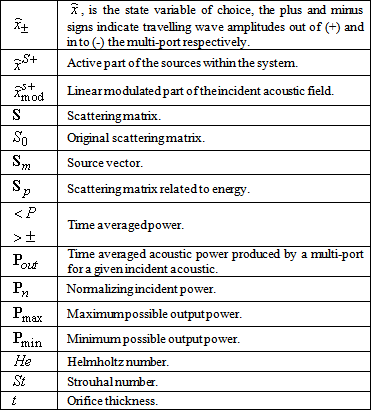### References

  Bodén H. and Åbom M., “Modelling of fluid machines as sources of sound in duct and pipe systems”, Acta Acustica (3), 1995, pp. 549-560.In article  Sabry Allam and Mats Åbom “Whistling Potential for Duct Components“. SAE Paper 2013-01-1889. May 20-23, 2013, Grand Rapids, Michigan, USA.In article  Radavich P., Selamet A. and Novak J. “A computational approach for ﬂow–acoustic coupling in closed side branches”, J. Acoust. Soc. Am. 109 (4), 2001, pp 1343-1353.In article CrossRef PubMed  Knutsson, M. and Bodén, H., “IC-Engine Intake Acoustic Source Data from Non-Linear Simulations”. SAE Technical Paper 2007-01-2209.In article  De Roeck W., Solntseva V. and W. Desmet (2008), “Numerical methodologies to predict the noise generation and propagation mechanisms in multiple expansion chambers”, AIAA (2008)-paper 2949.In article  Kierkegaard A., Boij S. and Efraimsson G. “A frequency domain linearized Navier-Stokes equations approach to acoustic propagation in flow ducts with sharp edges”, J. Acoustical Soc. of America (127) 2010, pp 710-719.In article  Aurégan Y. and Starobinski R., “Determination of acoustical dissipation/production potentiality from the acoustical transfer function of a multiport”, Acta Acustica, vol. 85, 1999, pp 788-792.In article  Åbom M., Allam S. and Boij S., “Aero-acoustics of flow duct singularities at low Mach number flows”, Vol. 1, AIAA, 2006, pp. 2687.In article  Testud P., Aurégan Y., Moussou P. and Hirschberg A., “The whistling potentiality of an orifice in a confined flow using an energetic criterion”, Journal of Sound and Vibration (325), 2009, pp 769-780.In article  Sattelmayer T. and Polifke W., “Assessment of methods for the computation of the linear stability of combustors”, Combustion Science and Technology (175), 2003, pp 453-476.In article  Karlsson M. and Åbom M., “Aeroacoustics of T-junctions an experimental study”, Journal of Sound and Vibration 329 (2010) 1793-1808.In article CrossRef  Kierkegaard A., Allam S., Efraimsson G. and Mats Å. (2012), “Simulations of whistling and the whistling potentiality of an in-duct oriﬁce with linear aeroacoustics” Journal of Sound and Vibration (331) 2012, pp 1084-1096.In article CrossRef  Kong, H., Woods, R., 1992, “Tuning of Intake Manifold of an Internal Combustion Engine Using Fluid Transmission. Line Dynamics”, International Congress & Exposition, SAE, February.In article  Åbom M. “Measurement of the Scattering-Matric of Acoustical Two-Ports”. Mechanical Systems and Signal Processing 5(2), 1991, pp 89-104.In article CrossRef  M. Karlsson and M. Åbom, “Aeroacoustics of T-junctions an experimental study”, Journal of Sound and Vibration 329 (2010) 1793-1808.In article CrossRef